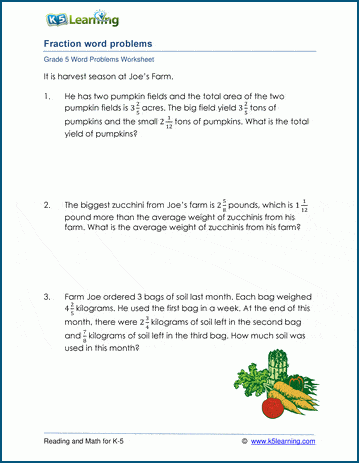# Add & subtract mixed numbers

## Fraction word problem worksheets: Add & subtract mixed numbers

Below are three versions of our grade 5 fractions worksheet with word problems involving adding and subtracting mixed numbers; problems involve both like and unlike denominators and sometimes more than two terms.  Some problems include superfluous data, forcing students to read and think about the questions, rather than simply recognizing a pattern to the solutions.  These worksheets are pdf files.## More word problem worksheets

Explore all of our math word problem worksheets, from kindergarten through grade 5.

## What is K5?

K5 Learning offers reading and math worksheets, workbooks and an online reading and math program for kids in kindergarten to grade 5.  We help your children build good study habits and excel in school.